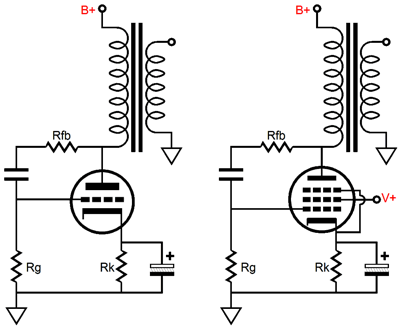<< March 2001 Tube CAD Journal

In fact, one way of looking at this topology is to see it as a means of making a pentode act more like a triode and making the triode act even more triode like. Let's review the basics. Normally, a pentode is extremely sensitive to variations in grid and screen voltage, but almost completely insensitive to variations in plate voltage, thus the pentode's high output impedance. The triode, on the other hand, is sensitive to plate voltage variations, as those variations will result in real variations in plate current. Put mathematically, the plate is 1/mu times as effective as the grid in controlling current flow through the triode (the cathode is [mu + 1] times as effective as the plate); and considering that a 300B has a mu of about 4, even being only one fourth as effective is something substantial.

The triode's plate, very much like a resistor, will resist an increase in plate voltage by increasing its current conduction and will resist an decrease in plate voltage by decreasing its current conduction; thus, the plate's opposition to voltage variations defines a negative feedback mechanism. In other words, the triode already enjoys a short, fast feedback loop from its plate to its cathode.

Applying a short, fast feedback loop to a pentode will bestow a much lower plate resistance to the pentode, while still retaining the pentode's wider useable current and voltage swing. Yes, it is a little like having one's cake and eating it, which was, if you will remember, the promise made by using an ultra-linear topology. (In many ways ultra-linear is a bestowing of triode like characteristics onto the pentode, while still preserving the pentode' features.)

However, nothing comes for free and the price to be paid for this enhanced performance is that transconductance will be needed to fuel the feedback mechanism. But before jumping to far ahead, let's start with the circuit's defining features and functioning.

### A Virtual Resistor

Beginning with the feedback loop, but in greater detail, imagine either a triode (say, a 300B) or a pentode (say, a 350B or EL34) whose grid was shorted to its plate via a coupling capacitor, as shown in the following schematic. As this circuit is now configured, it is not an amplifier, instead and in terms of AC signals, it is a virtual resistor of sorts, and a low valued resistor at that. For example, a +1 volt pulse in plate voltage will be immediately relayed to the grid, which will prompt the tube's entire transconductance to counter this voltage by increasing the tube's conduction.Virtual resistors made out of a triode and a pentode

In addition, the 300B's plate resistance will also buck this +1 volt pulse by making its contribution to the increase in the 300B's current conduction. For example, a +1 volt plate voltage pulse finds a 5.5 mA increase in current conduction from the +1 volt grid voltage pulse times the transconductance (5.5 mA/V) of the 300B, to which must be added to the 1.4 mA increase in current conduction from the  300B's rp (700 ohms). In other words, any voltage source used to increase the plate voltage by 1 volt would have to be able to also source 6.9 mA of current into the plate to sustain that +1 volt increase. Again, in other words, the 6.9 mA increase in current would be identical to the increase in current a 145 ohm impedance would give rise to when presented with a +1 voltage pulse (R = 1 / I or I = V / R). Adding the two sources of changes in current conduction together into one formula yields the effective transconductance of the triode:

Gm´ =  (mu + 1) / rp.

Considering that, in tube terms, the 300B's stock plate impedance of 700 ohms is already extremely low, a rp of 145 ohms is spectacularly low.

Had we used a 350B (a pentode of the beam power type) in the last example, the results would resemble those of the 300B. While this pentode does not have the highest plate resistance (37,500 ohms) of pentodes, but its rp is still hugely greater than the 300B's. It does, however, have a fair amount of transconductance (8.3  mA/V). So while its plate will make little contribution to the increase in current conduction (0.0266 mA), its grid acting on the tube's transconductance will. Thus, a +1 volt pulse on the plate equals a total of 8.3266 mA increase in current conduction. And thus, the 350B effectively equals a 120 ohm impedance in this circuit.